# A Finite Math Companion in R Markdown

## Foreword

The disciplines of statistics and probability build upon elementary subjects in the field of finite mathematics. This guide compiles many frequently used formulas, visualizations and theorems in this field of study into R programming language and presents these concepts in a written, textbook-style guide. In this guide, each chapter contains a different general subject matter, and contains a variety of formulas that can be called as a function. These functions may be called in a series, or nested into other formulas, to generate useful calculations and visualizations.

Put simply, finite mathematics is a collection of idioms, principles, and theorems concerning generally whole numbers. This includes a variety of subjects “other than calculus”. The subjects of matrix operations, linear programming, and set theory are key aspect of finite maths - and these subjects can act as the basis for many statistical analysis projects. Understanding how to define a sample space and determine expected value underpins all calculations of probability.

Many important financial formulas are also considered germane to the study of finite math, providing formulas to solve problems in everyday finance. Subjects of interest, annuities, sinking funds, amortization, and present value can help answer questions like: How much will I pay in total for a car or home loan?; How much do I need to save to reach a specified retirement goal?

We will also explore some real-world applications of these concepts, such as: determining how the quantity of a set of ingredients needed to have to make a certain quantity of a final recipe; to allocate resources needed to maximize profits, or applying the Leontief input-output model to extend this idea to the entire economy - determining the “true cost” of bringing a quantity of a resource to market, in cases when that resource (like fuel) may require some of itself in the process of producing or transporting of that resource.

This paper is not intended as a comprehensive guide to the field of finite mathematics; rather, it is intended to show step-by-step how the R programming language can be used as a tool to complete finite mathematics problems, and to highlight the capabilities of R for matrix operations, visualization of sets, and calculation of expected value. We will explore how various code constructs can be used to quickly define sample spaces, run Bernoulli trials, and generate randomized and specified elements for set-wise comparison. We will explore R’s ability to analyze sets for intersections, unions, complements and subsets, as well as to test and confirm that the sets conform to mathematical laws and counting formulas.

By carefully considering the problem, and constructing sample spaces where each outcome is equally likely, the expected value can be estimated, and the probability of any given outcome can be calculated using Bayesian statistics and rules about conditional probability under conditions of dependence, independence, or mutual exclusivity.

R and R Markdown provide a powerful platform for general statistical programming. Visualization capabilities in R are enhanced through the “Grammar of Graphics” as implemented through the GGPlot2 and GGForce packages. Data manipulation functions like select, mutate, and summarize are added by the dplyr package, enabling more convenient and readable R language constructs, using the pipe %>% operator. This operator can help your write intuitive syntax in R that resembles common structured query languages like SQL.

This document will not provide basic instruction in R. It is assumed that the reader has some familiarity with the R language, syntax patterns, installation, and configuration. If this is your first introduction to R, please check out the appendix to learn more about the basics of this language and installing the RStudio programming environment. The Rmd files (which this document was written in) may be downloaded from github, enabling the reader to change any portion of this document and customize the word problems to cover different applied subjects.

This guide is intended for finance professionals, statisticians, data scientists, math teachers, students*, or anyone else who needs to generate, visualize, apply, or solve finite math problems.

• Note to students: Many of these formulas can be used to solve finite math challenges; but you should avoid using this guide to answer questions on exams or as a shortcut to doing the hard work of understanding this important subject. The only way to the reach the goal of deep understanding, is by doing the work by hand. So please, do yourself a favor, and learn these concepts on pencil and paper before you use this guide. Your instructors will always want to see your work, and this guide won’t help you with that!

This Rmarkdown file will use several packages as defined below. This code will load the packages only of they are not already installed. These packages will be loaded into the local scope via the library functions.

``````if(!require(pracma)) install.packages("pracma", repos = "http://cran.us.r-project.org")
if(!require(dplyr)) install.packages("dplyr", repos = "http://cran.us.r-project.org")
if(!require(ggplot2)) install.packages("ggplot2", repos = "http://cran.us.r-project.org")
if(!require(ggforce)) install.packages("ggforce", repos = "http://cran.us.r-project.org")
if(!require(gtools)) install.packages("gtools", repos = "http://cran.us.r-project.org")
if(!require(matlib)) install.packages("matlib", repos = "http://cran.us.r-project.org")
if(!require(MASS)) install.packages("MASS", repos = "http://cran.us.r-project.org")
if(!require(kableExtra)) install.packages("kableExtra", repos = "http://cran.us.r-project.org")
if(!require(RcppAlgos)) install.packages("RcppAlgos", repos = "http://cran.us.r-project.org")
if(!require(latex2exp)) install.packages("latex2exp", repos = "http://cran.us.r-project.org")

library(pracma)
library(dplyr)
library(ggplot2)
library(ggforce)
library(gtools)
library(matlib)
library(MASS)
library(kableExtra)
library(RcppAlgos)
library(latex2exp)

options(scipen=999)``````

## Linear Equations

This section demonstrates some ways to perform basic visualizations that we will build upon later. Many applied problems in finite math involve drawing lines on a plane. GGplot makes this type of visualization fast, easy and convenient. When you pass data frames into a ggplot object and set the x and y variables, ggplot automatically determines the range of each axis and generates visual output to the graphics device in R. These plots can be rendered to the screen or saved in a variable that you add additional layers to.

### Graphing 2 Points on a Plane

Create two points and build a simple plot with just those 2 points at {0,0} and {10,10}. We will make them size 3 to ensure they are easy to see.

``````x_points <- c(0,10)
y_points <- c(0,10)
coords <- data.frame(x=x_points,y=y_points)

simple_plot <- ggplot(data=coords, aes(x=x, y=y)) +
geom_point(size=3)
simple_plot``````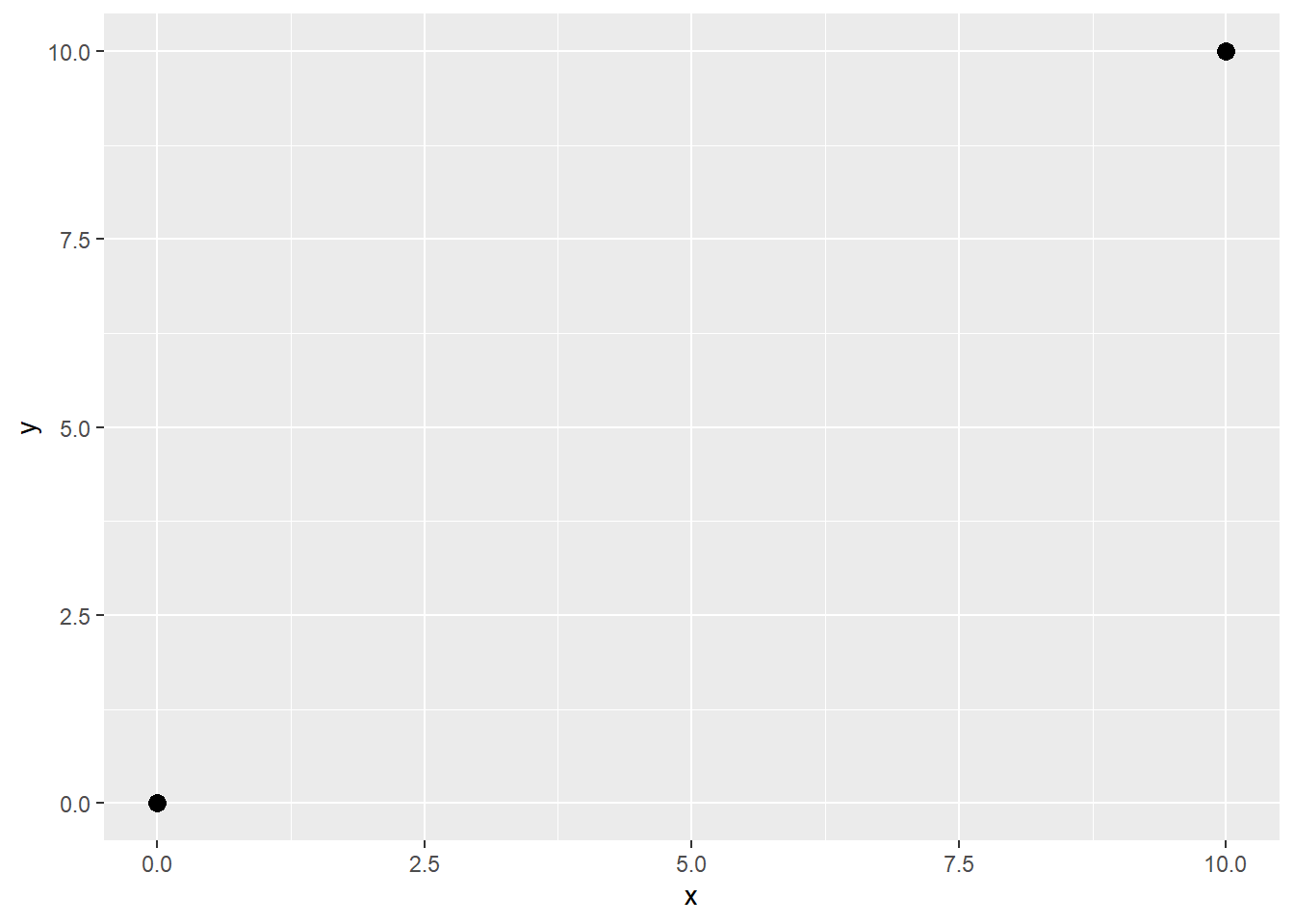Note how GGPlot has automatically made the plot area appropriate to the location of the two points.

### Graphing a Line between 2 Points

Create two points and build draw a line with just 2 points at {0,0} and {10,10}

To add a line between these two points, simply add a geom_line object. In the GGPlot syntax - the aes() argument defines where the data is coming from (coords) and which fields in the dataset should correspond to x/y points drawn on the chart.

``````x_points <- c(0,10)
y_points <- c(0,10)
coords <- data.frame(x=x_points,y=y_points)

simple_plot <- ggplot(data=coords, aes(x=x, y=y)) +
geom_point(size=3) +
geom_line()
simple_plot``````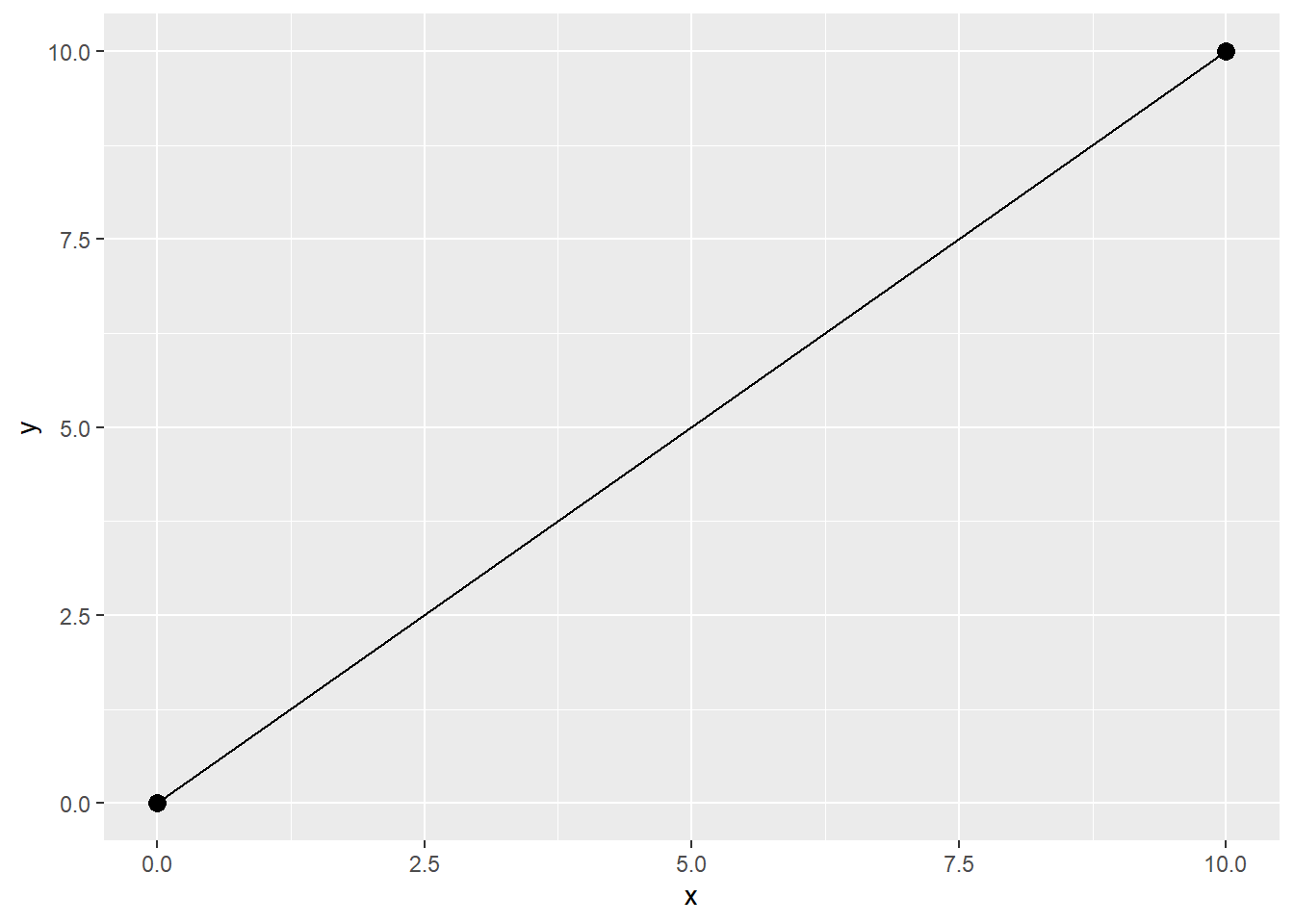### Slope of a Line

Our first equation will be to calculate the slope of a line. For each formal equation we create, we will create a function that encapsulates that function.

Calculate the slope of a line and display it on a chart as an annotation:

``````#x/y points
x_points <- c(0,8)
y_points <- c(0,6)
coords1 <- data.frame(x=x_points,y=y_points)

#slope of a line equation
slope_line <- function(coords1){
m <- (coords1\$y - coords1\$y) / (coords1\$x - coords1\$x)
m
}

#run the function and set the output to variable m
m <- slope_line(coords1)

#plot
simple_plot <- ggplot(data=coords1, aes(x=x, y=y)) +
geom_point(size=3) +
geom_line() +
annotate("text", x=3,y=3,label = paste("Slope:",m))
simple_plot``````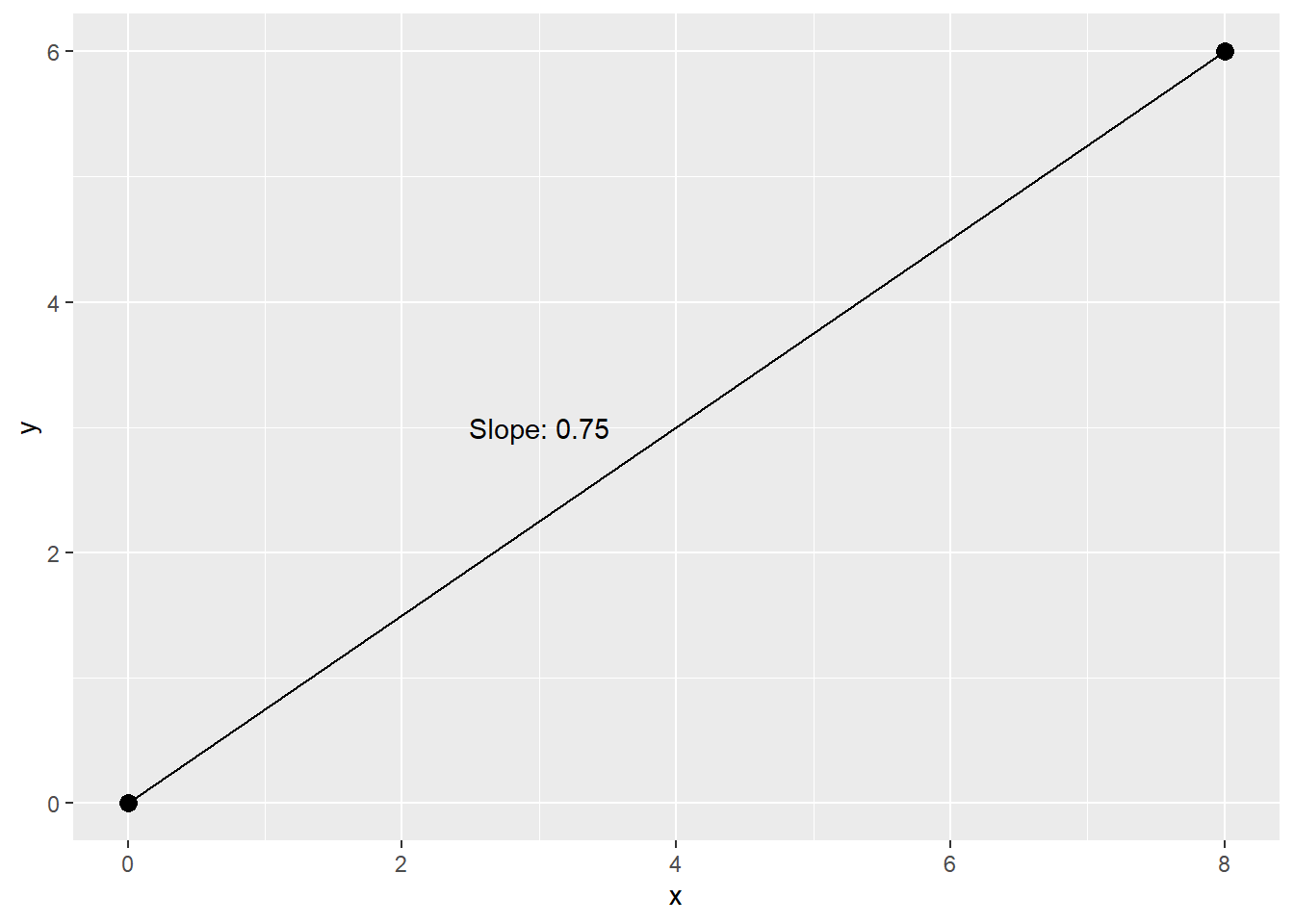### Graphing a Line with geom_smooth

This is a variant of the above line graphs. the geom_smooth produces a linear regression line between n points. This will draw a line, based on your points, using a model of your choosing. We will start with a linear model with only 2 observations - this will produce a straight line betwee n these two points.

This object will be important in later chapters as we explore plots that contain many points. In this section we are introducing the coord_cartesian object. This object will “zoom in” on a portion of the area, without dropping any points. This is similar to the scale object which sets the scale of the area. The difference is that scale removes observations outside the visible scale limits.

Adding the fullrange=TRUE tells GGplot to extend the line into an infinite space, filling the chart area from edge to edge. This is appropriate for linear programming formulas since the line is not finite, and we are interested in knowing where 2 or more lines intersect, without defining a start or end point of the lines. The starting and ending points of the line don’t exist - all that exists is a set of observations. A line should follow the slope of the known observations without terminating at any specified location in an x/y coordinate space.

``````#x/y points
x_points <- c(2,6)
y_points <- c(2,8)
coords1 <- data.frame(x=x_points,y=y_points)

#set bounds of focus area
upper_bound <- 10
lower_bound <- -10
left_bound <- -10
right_bound <- 10

#set limits to extend beyond the bounds
lower_bound_f <- ifelse(lower_bound>0,0,lower_bound)
left_bound_f <- ifelse(left_bound>0,0,left_bound)
upper_bound_f <- ifelse(upper_bound<0,0,upper_bound)
right_bound_f <- ifelse(right_bound<0,0,right_bound)

#plot
simple_plot <- ggplot() +
geom_point(data=coords1, size=3, aes(x=x, y=y)) +
xlim(left_bound_f*2, right_bound_f*2) +
ylim(lower_bound_f*2, upper_bound_f*2) +
geom_smooth(data=coords1, aes(x=x, y=y),method="lm",fullrange=TRUE,color="black") +
geom_hline(yintercept=0) +
geom_vline(xintercept=0) +
coord_cartesian(xlim = c(left_bound, right_bound),
ylim = c(lower_bound, upper_bound),
expand = FALSE)
simple_plot``````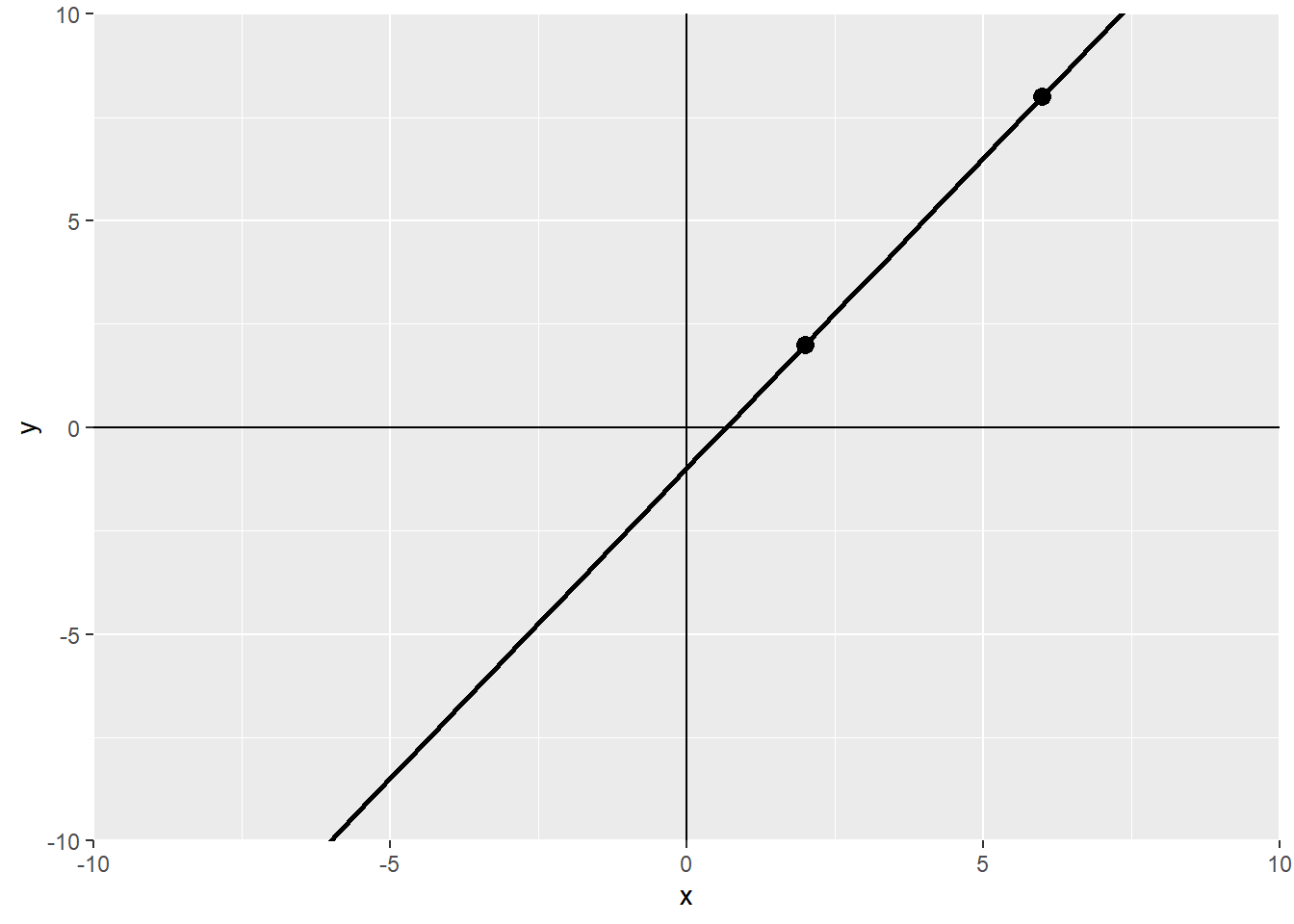### Graphing a Linear Equation with 3 or more Points with geom_smooth

This geom_smooth produces a linear regression line between 3 points. The shaded area represents the standard error of this regression line using a 95% confidence interval by default.

``````#x/y points
x_points <- c(0,3,5,8)
y_points <- c(0,3,3,6)
coords1 <- data.frame(x=x_points,y=y_points)

#plot
simple_plot <- ggplot(data=coords1, aes(x=x, y=y)) +
geom_point(size=3) +
geom_smooth(method="lm")
simple_plot``````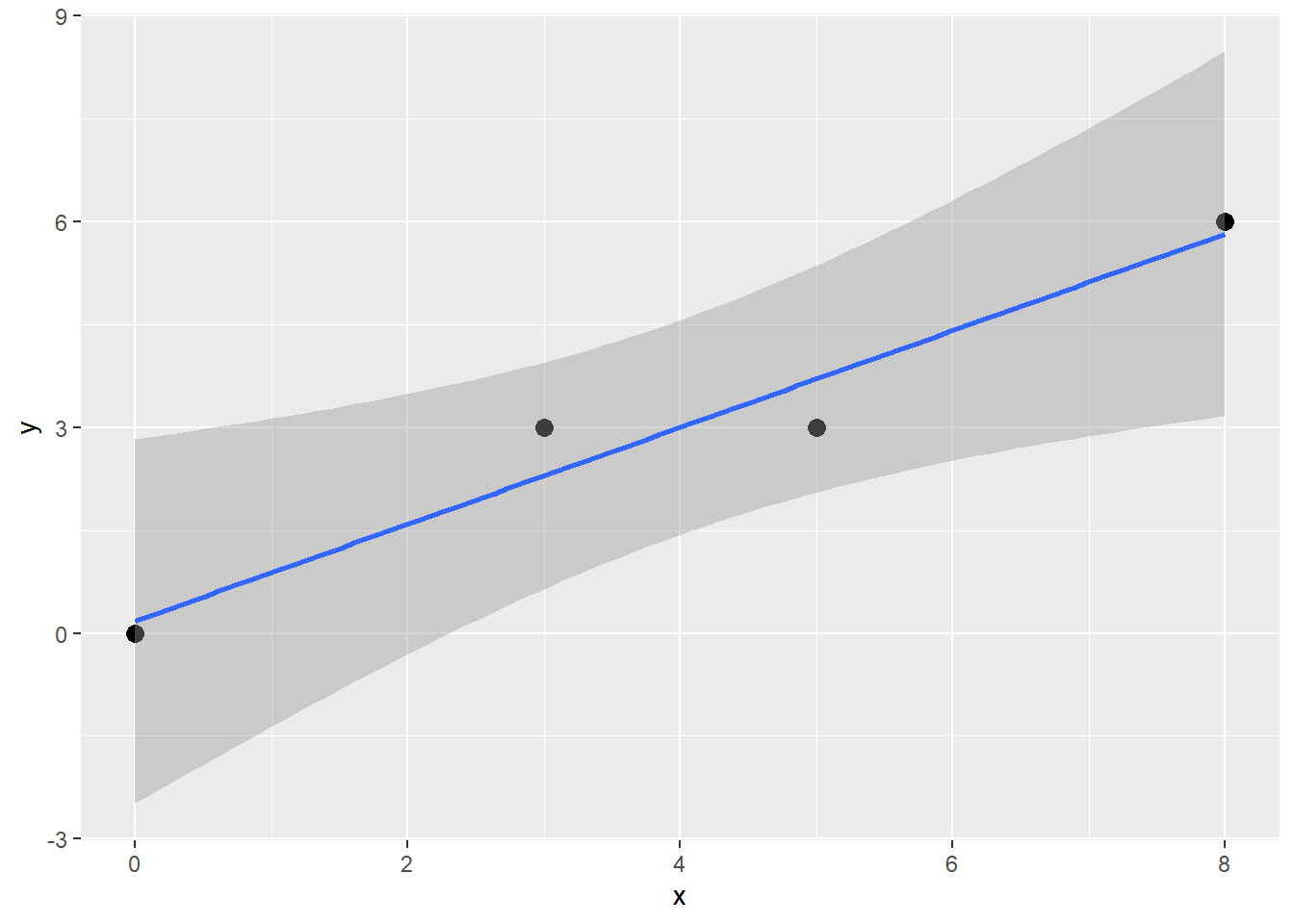The geom_smooth may also be used to draw a line using a loess kernal method to produce a curved regression line between 3 or more points. Drawing a smoothed curve is not strictly considered part of the discipline of finite maths, but it will be important in later chapters on probability and statistics.

``````#x/y points
x_points <- c(0,3,5,8)
y_points <- c(0,3,3,6)
coords1 <- data.frame(x=x_points,y=y_points)

#plot
simple_plot <- ggplot(data=coords1, aes(x=x, y=y)) +
geom_point(size=3) +
geom_smooth(method="loess")
simple_plot``````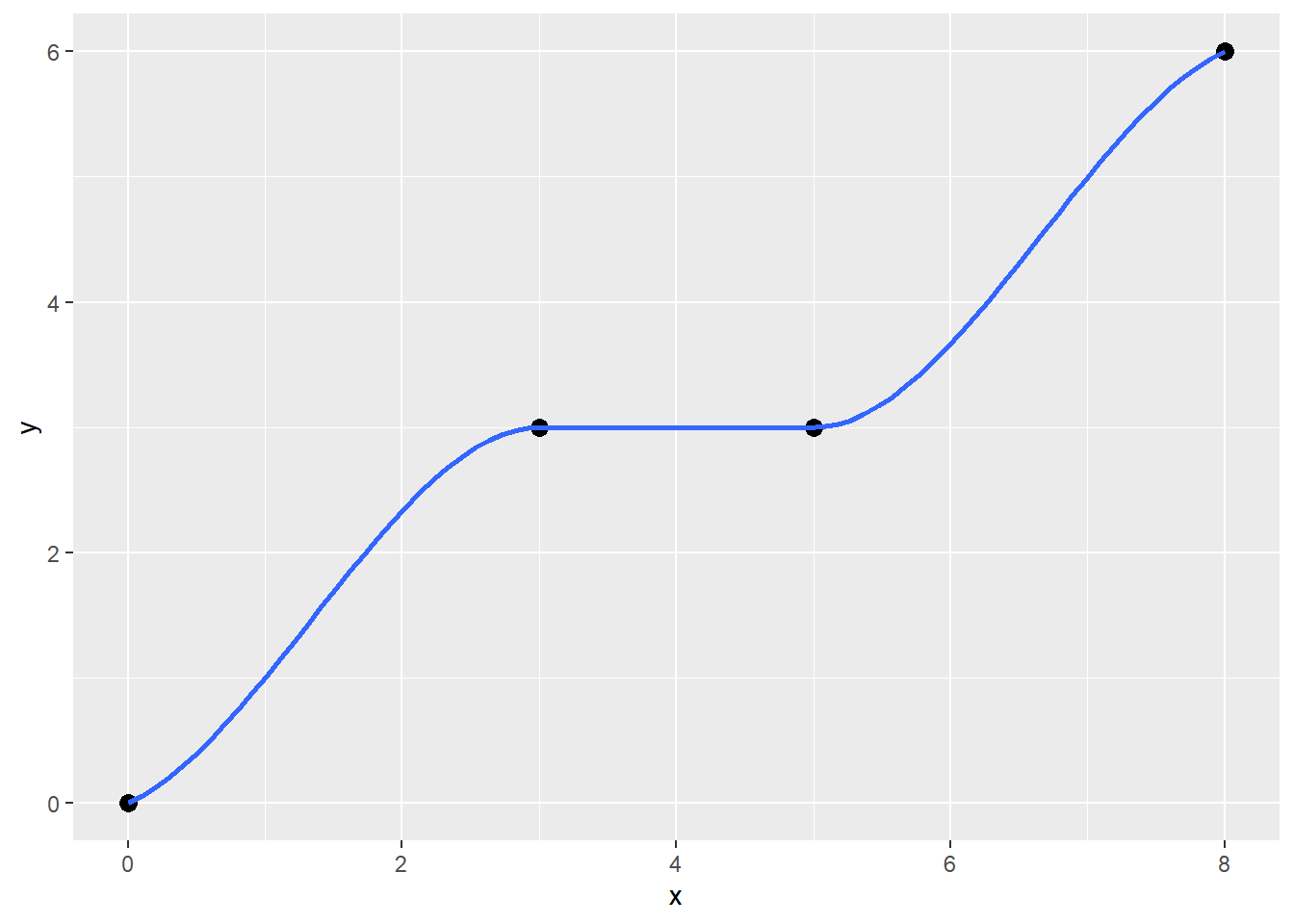### Graphing a line with arrows with geom_segment

Another way to represent an infinite line that continues into an endless space, is to use the geom_segment object. This geom_segment object produces a line between 2 points with an arrowhead on the end. You can add two lines that are both covering the same x/y space, but going opposite directions, with points size set to 0, to simulate the appearance of an infinite line as it might appear in a traditional math text. This approach is a bit cumbersome!

``````x_points <- c(0,10)
y_points <- c(0,10)
coords <- data.frame(x=x_points,y=y_points)

#plot
simple_plot <- ggplot(data=coords, aes(x=x, y=y)) +
geom_point(size=0) +
geom_segment(x=coords\$x,
xend=coords\$x,
y=coords\$y,
yend=coords\$y,
arrow = arrow(length = unit(.2, 'cm'))) +
geom_segment(x=coords\$x,
xend=coords\$x,
y=coords\$y,
yend=coords\$y,
arrow = arrow(length = unit(.2, 'cm')))
simple_plot``````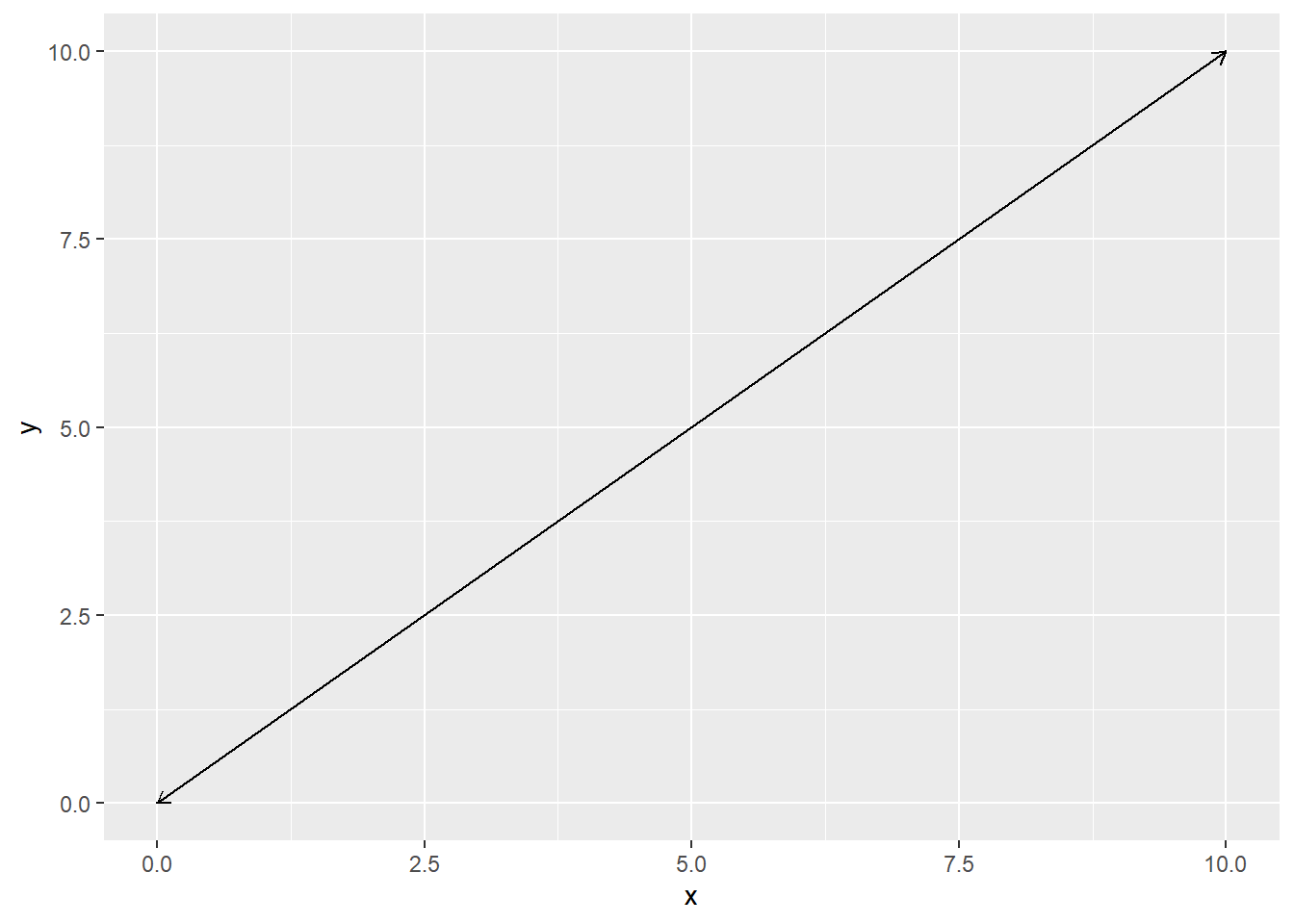### Graphing 2 Lines

Create 2 sets of 2 points and draw a line between them.

In this example, the two equations are being treated as 2 separate data frames. This enables us to pass these into the ggplot objects data parameter for each element we want to draw. We are also adding some text to show the {x,y} coordinates of each point.

Tech Note: The data parameter in this example has moved out of the ggplot() object, and into the individual geom_ elements. This is because this ggplot constructor does not have one “primary” data set, but two. Any settings specified in the ggplot() constructor including data or aes mappings, will be inherited by child objects added to the plot via the + operator. Further, any parameters set in the child object will override any setting in the ggplot constructor. If you are familiar with CSS style sheets - GGplot has similar inheritance structures. You set styles in the GGplot that cascade from a less specific element (GGplot) to a more specific element like geom_line, geom_text, etc.

``````# points
x_points <- c(0,10)
y_points <- c(0,4)
coords1 <- data.frame(x=x_points,y=y_points)

x_points <- c(0,6)
y_points <- c(8,0)
coords2 <- data.frame(x=x_points,y=y_points)

m1 <- round(slope_line(coords1),2)
m2 <- round(slope_line(coords2),2)

multi_plot <- ggplot() +
geom_text(data=coords1, aes(x=x, y=y, label=paste("{",x,",",y,"}")),color="black") +
geom_point(data=coords1, aes(x=x, y=y),color="blue") +
geom_line(data=coords1, aes(x=x, y=y),color="blue") +
annotate("text", x=9,y=3,color="blue",label = paste("Slope:",m1)) +
geom_text(data=coords2, aes(x=x, y=y, label=paste("{",x,",",y,"}")),color="black") +
geom_point(data=coords2, aes(x=x, y=y),color="darkgreen") +
geom_line(data=coords2, aes(x=x, y=y),color="darkgreen") +
annotate("text", x=2,y=6,color="darkgreen",label = paste("Slope:",m2))
multi_plot``````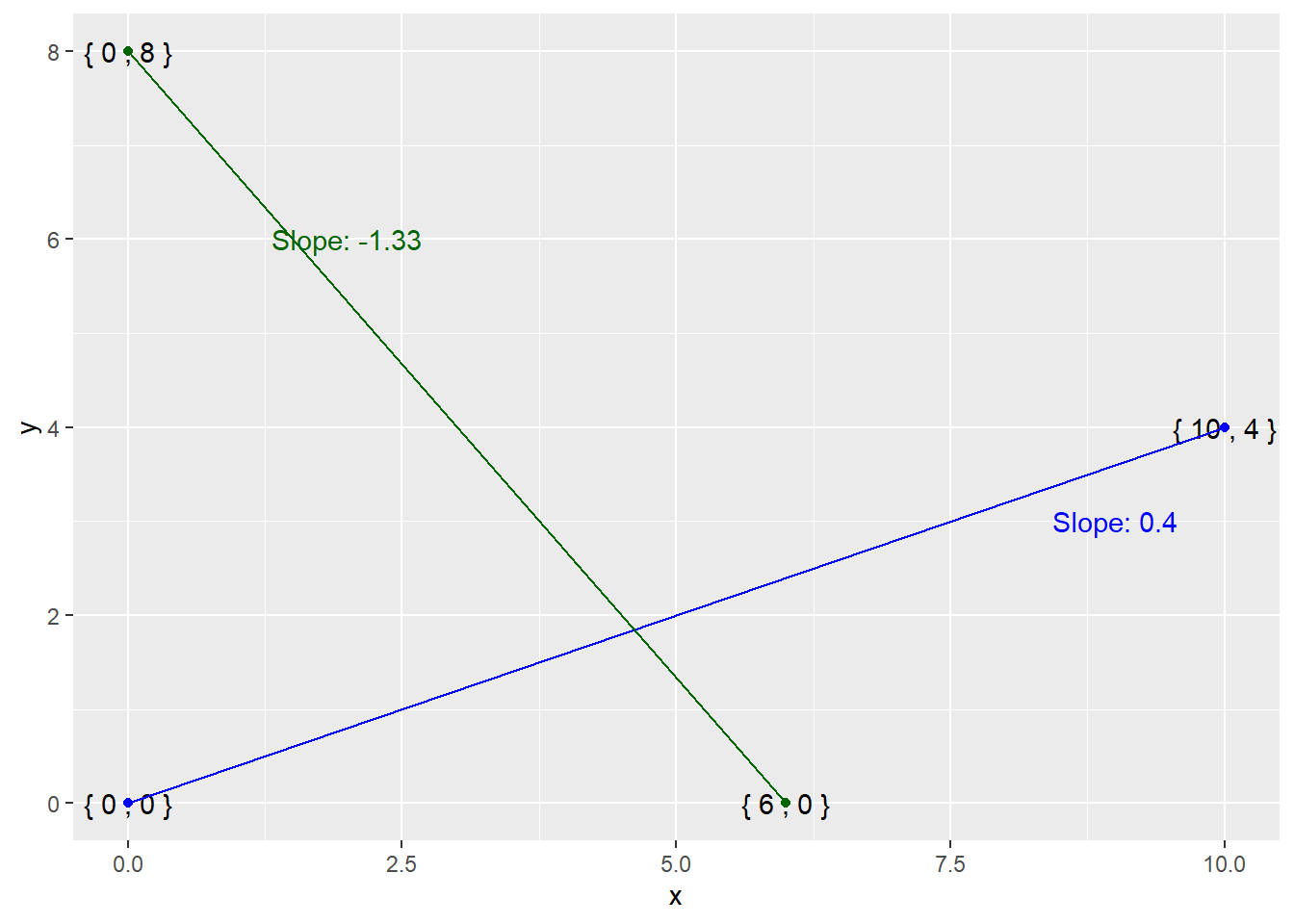In the chart above, the slope of the blue line is a positive number since x and y axis are increasing together - visually the line is rising from left to right. The slope of the green line is a negative number, since the y axis is decreasing as the x axis is increasing.

### Check Intersection of 2 Lines

This function will to determine if 2 lines intersect by checking the slope of each line using our slope_line function on both sets of coordinates. If the slope is not equal - the lines must intersect (eventually). The function will return TRUE if the lines intersect.

Tech Note: This function assumes that the lines continue forever in both directions. It is only checking that the slope of the two lines is not equal, not detecting if any two segments overlap.

``````#x/y points
x_points <- c(0,10)
y_points <- c(0,4)
coords1 <- data.frame(x=x_points,y=y_points)

x_points <- c(0,6)
y_points <- c(8,0)
coords2 <- data.frame(x=x_points,y=y_points)

#check if the slopes intersect
check_intersect <- function(coords1,coords2){
m1 <- slope_line(coords1)
m2 <- slope_line(coords2)
is_intersecting <- ifelse(m1 == m2,FALSE,TRUE)
is_intersecting
}

#run intersect check
intersects <- check_intersect(coords1,coords2)

#plot
multi_plot <- ggplot() +
geom_point(data=coords1, aes(x=x, y=y),color="blue") +
geom_line(data=coords1, aes(x=x, y=y),color="blue") +
geom_point(data=coords2, aes(x=x, y=y),color="green") +
geom_line(data=coords2, aes(x=x, y=y),color="green") +
annotate("text", x=5,y=5,label = paste("Intersecting?:",intersects))
multi_plot``````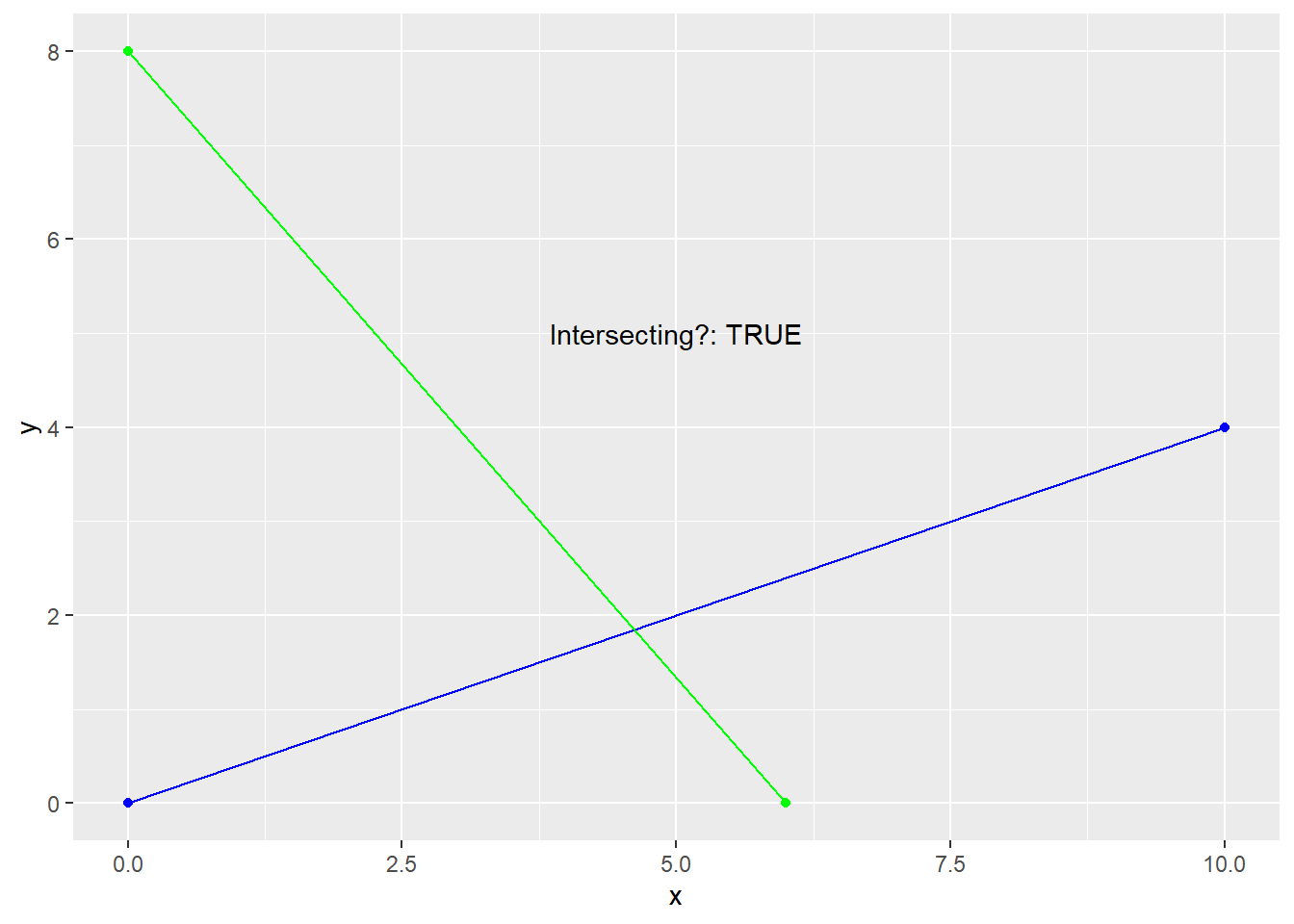If the lines do not intersect, as shown below, they must be parallel lines.

``````#x/y points
x_points <- c(0,10)
y_points <- c(10,0)
coords1 <- data.frame(x=x_points,y=y_points)

x_points <- c(0,9)
y_points <- c(9,0)
coords2 <- data.frame(x=x_points,y=y_points)

#run intersect check
intersects <- check_intersect(coords1,coords2)

#plot
multi_plot <- ggplot() +
geom_point(data=coords1, aes(x=x, y=y),color="blue") +
geom_line(data=coords1, aes(x=x, y=y),color="blue") +
geom_point(data=coords2, aes(x=x, y=y),color="green") +
geom_line(data=coords2, aes(x=x, y=y),color="green") +
annotate("text", x=6,y=6,label = pas``````